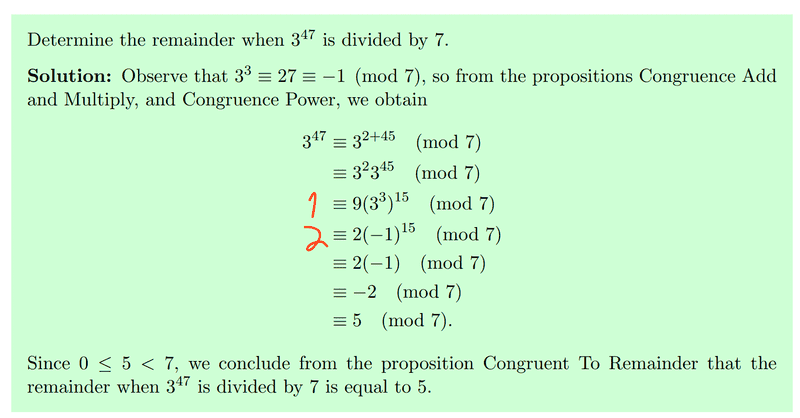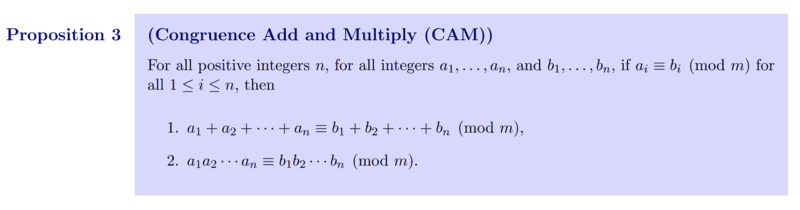# (Elementary number theory) Understanding congruence and modulus

• I
• Leo Liu

#### Leo Liu

TL;DR Summary
N/AIn this question, how does the step marked with 1 become the step marked with 2? I can see that the transitivity property of congruence is used, but I don’t know what exactly is going on here. Can someone please explain? Also at which step is Congruence Add and Multiply used?

Thanks.

Preposition used:Isn’t 9 mod 7 the value 2?

Similarly for 3^3 is 27 which is one less than 28.

what rule would they be using to do this?

Isn’t 9 mod 7 the value 2?

Similarly for 3^3 is 27 which is one less than 28.
Yes. But I just don't understand how the theorem is applied here.

The theorem says the modulo N of a sum or product of a list of numbers is the same as the sum or product of the modulos of those numbers, right?

•Leo Liu
The theorem says the modulo N of a sum or product of a list of numbers is the same as the sum or product of the modulos of those numbers, right?
Right. But I am afraid that I still cannot see why as the congruence equations are not in the same form as the preposition.

Right. But I am afraid that I still cannot see why as the congruence equations are not in the same form as the preposition.
What does that mean?

We have ##9 = 2 + 7##. Therefore ##9 \equiv 2 \ (mod \ 7)##. Therefore we may replace ##9## by ##2## in any equation modulo ##7##. That's the idea.

•Leo Liu
The proposition says that if ##a_1\equiv a_2 \; (mod\; m)## and ##b_1\equiv b_2 \; (mod\; m)##, then you have ##a_1b_1\equiv a_2b_2 \; (mod\; m)##.

In the example it is applied to ##9 \equiv 2 \; (mod\; 7)## and ##3^3 \equiv -1 \; (mod\; 7)##.

•Leo Liu
What does that mean?

We have ##9 = 2 + 7##. Therefore ##9 \equiv 2 \ (mod \ 7)##. Therefore we may replace ##9## by ##2## in any equation modulo ##7##. That's the idea.
Thanks. You answer helped me understand it intuitively. Yet I still don't know how the congruence add and multiply is applied to this step.

The proposition says that if ##a_1\equiv a_2 \; (mod\; m)## and ##b_1\equiv b_2 \; (mod\; m)##, then you have ##a_1b_1\equiv a_2b_2 \; (mod\; m)##.

In the example it is applied to ##9 \equiv 2 \; (mod\; 7)## and ##3^3 \equiv -1 \; (mod\; 7)##.
This makes sense, but could you explain why this expression still holds when a1 and b1 is followed by (mod m)?

Thanks. You answer helped me understand it intuitively. Yet I still don't know how the congruence add and multiply is applied to this step.
Proposition 3 that you quoted in your OP is a formal way to say you can replace a number by any other number of modular equivalence in products and sums. That's what it means - and Proposition 3 is that idea written out formally.

•Leo Liu